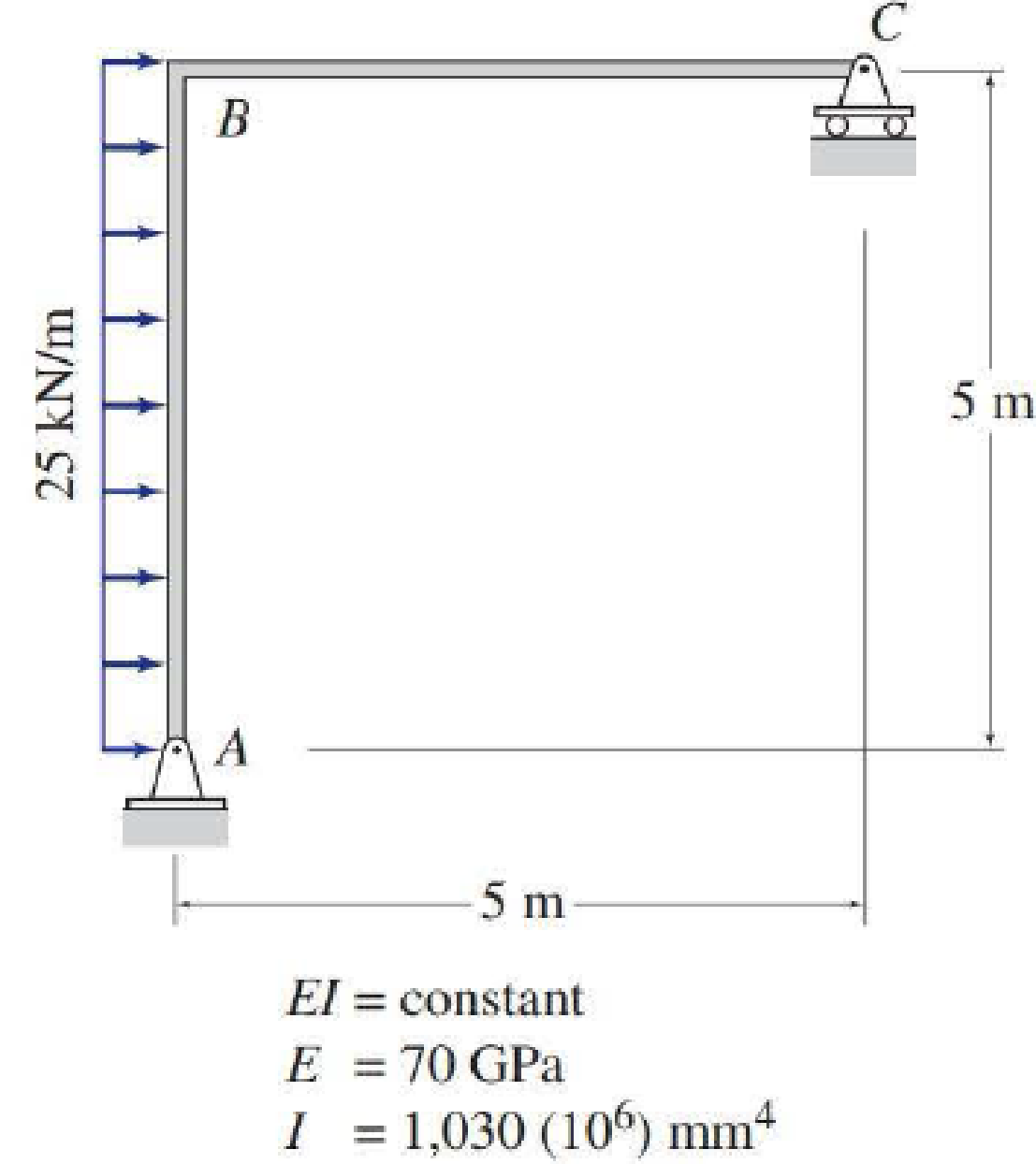# Use the virtual work method to determine the horizontal deflection at joint C of the frame shown.

#### Solutions

Chapter
Section
Chapter 7, Problem 38P
Textbook Problem
106 views

## Use the virtual work method to determine the horizontal deflection at joint C of the frame shown.To determine

Find the horizontal deflection at joint C of the frame using virtual work method.

### Explanation of Solution

Given information:

The frame is given in the Figure.

The value of E is 70 GPa and I is 1,030(106)mm4.

Apply the sign conventions for calculating reactions, forces and moments using the three equations of equilibrium as shown below.

• For summation of forces along x-direction is equal to zero (Fx=0), consider the forces acting towards right side as positive (+) and the forces acting towards left side as negative ().
• For summation of forces along y-direction is equal to zero (Fy=0), consider the upward force as positive (+) and the downward force as negative ().
• For summation of moment about a joint is equal to zero (Matapoint=0), consider the clockwise moment as negative and the counter clockwise moment as positive.

Calculation:

Consider the real system.

Draw a diagram showing all the given real loads acting on it.

Let the bending moment due to real load be M.

Sketch the real system of the frame as shown in Figure 1.

Find the reactions at the supports A and C:

Summation of moments about A is equal to 0.

MA=0Cy(5)25(5)(52)=0Cy=62.5kN

Summation of forces along y-direction is equal to 0.

+Fy=0Ay+Cy=0Ay+62.5=0Ay=62.5kN

Summation of forces along x-direction is equal to 0.

+Fx=0Ax+25(5)=0Ax=125kN

Consider the virtual system.

Draw a diagram of frame without the given real loads. For horizontal deflection apply unit load at the joint in the horizontal direction.

Let the bending moment due to virtual load be Mv.

Sketch the virtual system of the frame with unit load at joint C as shown in Figure 2.

Find the reactions at the supports A and C:

Summation of moments about A is equal to 0.

MA=0Cy(5)1(5)=0Cy=1kN

Summation of forces along y-direction is equal to 0.

+Fy=0Ay+Cy=0Ay+1=0Ay=1kN

Summation of forces along x-direction is equal to 0.

+Fx=0Ax+1=0Ax=1kN

Find the equations for M and Mv for the 2 segments of the frame as shown in Table 1.

 Segment x-coordinate M (k-ft) Mv (k-ft) Origin Limits (ft) AB A 0−5 125x−12.5x2 1x CB C 0−5 62.5x 1x

Find the horizontal deflection at C using the virtual work expression:

1(ΔC)=0LMvMEIdx (1)

Rearrange Equation (1) for the limits 05 and 05 as follows

### Still sussing out bartleby?

Check out a sample textbook solution.

See a sample solution

#### The Solution to Your Study Problems

Bartleby provides explanations to thousands of textbook problems written by our experts, many with advanced degrees!

Get Started

Find more solutions based on key concepts
The U.S. Bureau of Labor Statistics produces data sets that show how the price of goods and services changes fr...

Engineering Fundamentals: An Introduction to Engineering (MindTap Course List)

What is the purpose of CTE or vocational education?

Precision Machining Technology (MindTap Course List)

Fundamentals of Information Systems

What is a stored procedure, and why is it particularly useful? Give an example.

Database Systems: Design, Implementation, & Management

5. Describe a data dictionary and list the types of information it contains.

Systems Analysis and Design (Shelly Cashman Series) (MindTap Course List)

What is a subquery?

A Guide to SQL

Connecting to a Projector Your boss asked you to give a presentation to your companys board of directors. When ...

Enhanced Discovering Computers 2017 (Shelly Cashman Series) (MindTap Course List)

If your motherboard supports ECC DDR3 memory, can you substitute non-ECC DDR3 memory?

A+ Guide to Hardware (Standalone Book) (MindTap Course List)

How can cutting torch tip seals be repaired?

Welding: Principles and Applications (MindTap Course List)